How Cheenta works to ensure student success?
Explore the Back-Story

# Points on a circle | AMC 10A, 2010| Problem No 22Try this beautiful Problem on Number theory based on Points on a circle from AMC 10 A, 2010. You may use sequential hints to solve the problem.

## Points on a circle - AMC-10A, 2010- Problem 22

Eight points are chosen on a circle, and chords are drawn connecting every pair of points. No three chords intersect in a single point inside the circle. How many triangles with all three vertices in the interior of the circle are created?

,

•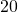•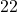•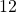•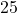•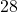Number theory

Combination

Circle

## Suggested Book | Source | Answer

Pre College Mathematics

#### Source of the problem

AMC-10A, 2010 Problem-22

#### Check the answer here, but try the problem first## Try with Hints

#### First Hint

To create a chord we have to nedd two points. Threfore three chords to create a triangle and not intersect at a single point , we have to choose six points.

Now can you finish the problem?

#### Second Hint

Now the condition is No three chords intersect in a single point inside the circle.Now we know that for any six points we pick, there is only 1 way to connect the points such that a triangle is formed in the circle's interior

Now Can you finish the Problem?

#### Third Hint

Therefore the required answer is=## Subscribe to Cheenta at Youtube

Try this beautiful Problem on Number theory based on Points on a circle from AMC 10 A, 2010. You may use sequential hints to solve the problem.

## Points on a circle - AMC-10A, 2010- Problem 22

Eight points are chosen on a circle, and chords are drawn connecting every pair of points. No three chords intersect in a single point inside the circle. How many triangles with all three vertices in the interior of the circle are created?

,

•••••Number theory

Combination

Circle

## Suggested Book | Source | Answer

Pre College Mathematics

#### Source of the problem

AMC-10A, 2010 Problem-22

#### Check the answer here, but try the problem first## Try with Hints

#### First Hint

To create a chord we have to nedd two points. Threfore three chords to create a triangle and not intersect at a single point , we have to choose six points.

Now can you finish the problem?

#### Second Hint

Now the condition is No three chords intersect in a single point inside the circle.Now we know that for any six points we pick, there is only 1 way to connect the points such that a triangle is formed in the circle's interior

Now Can you finish the Problem?

#### Third Hint

Therefore the required answer is=## Subscribe to Cheenta at Youtube

This site uses Akismet to reduce spam. Learn how your comment data is processed.

### Knowledge Partner